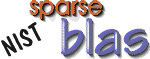# Sparse Basic Linear Algebra Subprograms (BLAS) Library

This page contains software for various libaries developed at NIST for the Sparse Basic Linear Algebra Subprograms (BLAS), which describes kernels operations for sparse vectors and matrices. The current distribution adheres to the ANSI C interface of the BLAS Technical Forum Standard. Older libraries corresponding to previous designs are also included for archival and historical purposes.

## Libraries for BLAS Technical Forum (BLAST) interfaces

• Download ANSI C++ implementation. This is an ANSI C++ implementation of the complete ANSI C specification. The distribution is quite small (less than 14 Kbytes) and it is meant as a starting for developing optimized and architecture-dependent version. (C++ was used, rather than C, as it has support for complex arithmetic and templates to facilitate to creation of various precision codes.) The library includes support for all four precision types (single, double precision real and complex) and Level 1, 2, and 3 operations.

• Download ANSI C implementation. This is an earlier ANSI C implementation which supports only double-precision operations.

## Original NIST Sparse BLAS (non-compliant interface)

Link to original NIST Sparse BLAS This is an implementation of a lower-level interface which was done before the BLAST Standard. (Actually, it was developed to help direct research while the BLAST standardizaiton process.) The operations are more general and support specific storage formats. It is much larger, but includes support for compressed-column/row storage formats, and block variants. Although it does not adhere to the BLAST Standard, the libraries are useful in their own right.

The BLAS Technical Forum Standard defines the following sparse matrix and vector operations:

## Sparse Vector (Level 1) Operations

` (1) r <-- op(x) * y sparse dot-product `
` (2) y <-- alpha * x + y sparse vector update`
` (3) x <-- y|x sparse gather`
` (4) x <-- y|x ; y|x = 0 sparse gather and zero`
` (5) y|x <-- x sparse scatter`
where r is a scalar, x is a sparse vector, y is a dense vector, y|x denotes the elements of y that are indexed by x.

## Matrix-Vector (Level 2) and Matrix-Matrix (Level 3) operations

` (6) y <-- alpha * op(A) * x + y matrix-vector multiply`
` (7) C <--- alpha * op(A) * B + C matrix-matrix multiply`
` (8) x <-- alpha * op(T) ^(-1) * x matrix-vector triangular solve`
` (9) B <-- alpha * op(T) ^(-1) * B matrix-matrix triangular solve`
where A is a sparse matrix, T is an triangular sparse matrix, x and y are dense vectors, B and C are (usually tall and thin) dense matrices, and op(A) is either A, the transpose of A, or the Hermitian of A.

Unlike their dense-matrix counterpart routines, the underlying matrix storage format is NOT described by the interface. Rather, sparse matrices must be first constructed before being used in the Level 2 and 3 computationalroutines.

There are various operations available for sparse matrix construction:
` (A) xuscr_begin() point (scalar) construction`
` (B) xuscr_block_begin() block construction`
` (C) xuscr_variable_block_begin() variable block construction`
` (D) xuscr_insert_entry() insert single (i,j) value`
` (E) xuscr_insert_entries() insert list of (i,j) values`
` (F) xuscr_insert_col() insert a sparse colum of values`
` (G) xuscr_insert_row() insert a sparse row of values`
` (H) xuscr_insert_clique() insert a clique of values`
` (I) xuscr_insert_block() insert a block of values`
` (J) xuscr_end() terminate matrix construction`
` (K) usgp() get matrix property`
` (L) ussp() set matrix property`
` (M) usds() destroy matrix`

Each BLAS routine (except for (K), (L), and (M) above) there is a specification for four floating point types (single precision, double precision, complex single precision, and complex double precision) hence, the above specification yields 79 Sparse BLAS routines.

See the following references for further information on  the Sparse BLAS specification, and  examples and discussion about the interface from some of the key members who worked on the standard:

(1) I. Duff, M. Heroux, R. Pozo, "An Overview of the Sparse Basic Linear Algebra Subprograms: The New Standard from the BLAS Technical Forum," ACM TOMS, Vol. 28, No. 2, June 2002, pp. 239-267.

(2) The BLAS Technical Forum Standard www.netlib.org/blas/blast-forum.

Development Status: Active Maintenance